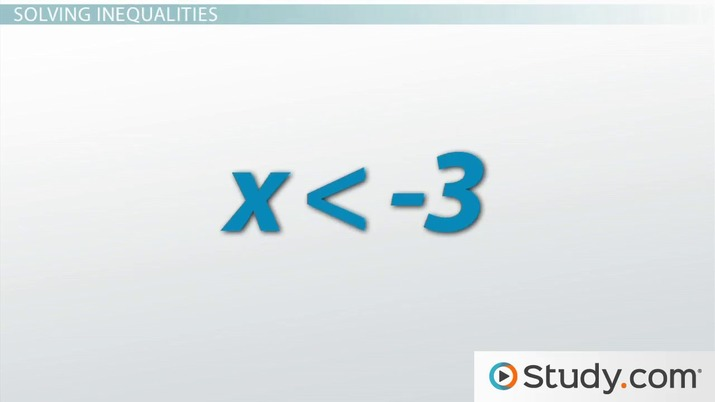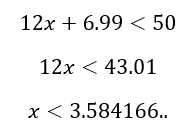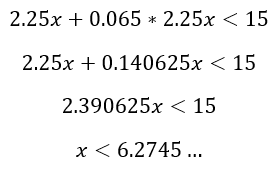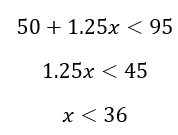# Less Than Symbol in Math: Problems & Applications

Lesson Transcript
Instructor
Jennifer Beddoe

Jennifer has an MS in Chemistry and a BS in Biological Sciences.

Expert Contributor
Kathryn Boddie

Kathryn has taught high school or university mathematics for over 10 years. She has a Ph.D. in Applied Mathematics from the University of Wisconsin-Milwaukee, an M.S. in Mathematics from Florida State University, and a B.S. in Mathematics from the University of Wisconsin-Madison.

In mathematics, the less-than symbol is used to represent the inequality of one number being less than another. Learn about the language of math, the symbols for greater than and less than, and explore how to solve inequalities through mathematical problems and applications. Updated: 09/07/2021

## The Language of Math

Mathematics is a language that uses many symbols. Learning all the symbols can be tricky, but it's critical for understanding what is being communicated, just like learning words and the rules for grammar is key to speaking and understanding a foreign language, like Spanish.An error occurred trying to load this video.

Try refreshing the page, or contact customer support.

Coming up next: What are 2D Shapes? - Definition & Examples

### You're on a roll. Keep up the good work!

Replay
Your next lesson will play in 10 seconds
• 0:01 The Language of Math
• 0:17 Greater Than or Less Than
• 1:15 Solving Inequalities
• 2:16 Applications
• 3:44 Lesson Summary
Save Save

Want to watch this again later?

Timeline
Autoplay
Autoplay
Speed Speed

## Greater Than or Less Than

When two things are the same in every way, they are said to be equal. When two values are not the same, there is an inequality. In math, problems most often center around equations of numbers that are either equal or unequal. If the equations or numbers are not equal, it stands to reason that one of them is bigger than the other.

The less-than symbol (< ) is used to signify that the number on the left is smaller, or less, than the number on the right. The greater-than symbol (>) is used to signify that the number on the left is larger, or greater, than the number on the right. The less-than and greater-than symbols are actually the same symbol, the direction of which is switched depending on whether the number on the left is larger or smaller.

You may remember learning to use these symbols with the aid of an alligator when you were younger. The alligator is hungry, and so he opens his mouth towards the bigger number.

## Solving Inequalities

The less-than symbol, as well as the greater-than symbol, can be used for more than just showing which number is larger than another. In fact, many inequalities require you to solve the problems on each side of the less-than symbol in order to determine the relative value of a variable. Here is an example of a math problem containing the less-than symbol:

3x + 2 < x - 4

Just like you would if there was an equal sign, you want to group like terms together. Subtracting an x from either side, we get:

2x + 2 < -4

Subtract a 2 from either side to get:

2x < -6

Finally, dividing by 2, we have:

x < -3

You can see that solving an inequality is very similar to solving an equation. One important difference is our solution is not a single value. In this example, our solution includes all values less than -3.

To unlock this lesson you must be a Study.com Member.

Applications in mathematics are often shown using word problems. Many students struggle with rewriting the words into math. In the following examples, students will practice translating real-world situations into inequalities and then will solve the inequalities.

## Examples

1) You want to purchase some DVDs from a website. Each DVD costs \$12.00, and shipping costs \$6.99. You have \$50.00 that you can spend. Write an inequality representing the situation and solve for the number of DVDs you can purchase.

2) Your favorite cereal is on sale at the grocery store for \$2.25 per box. Sales tax is 6.5%, and you have \$15.00. Write an inequality representing the situation and solve for the number of boxes you can buy.

3) A car rental costs \$50.00 plus an additional \$1.25 per mile driven. If you want to spend less than \$95.00 for a rental, write an inequality and solve it to find the number of miles you can drive.

## Solutions

1) If x stands for the number of DVDs you can buy, we can write the inequality 12x + 6.99 < 50, meaning that 12 dollars multiplied by the number of DVDs plus the shipping cost of \$6.99 must be less than \$50.00. To solve the inequality, subtract 6.99 from both sides and divide by 12.So you can buy at most 3 DVDs.

2) If x stands for the number of boxes of cereal, we can write 2.25x + 0.065 * 2.25x < 15. The sales tax of 6.5% is calculated by multiplying the cost of x boxes of cereal by 0.065. Then, simplifying and solving the inequality:So you can buy at most 6 boxes of cereal.

3) If x stands for the number of miles driven, then the car rental can be represented by 50 + 1.25x < 95. Solving this inequality, we have:So you must drive less than 36 miles.

### Register to view this lesson

Are you a student or a teacher?